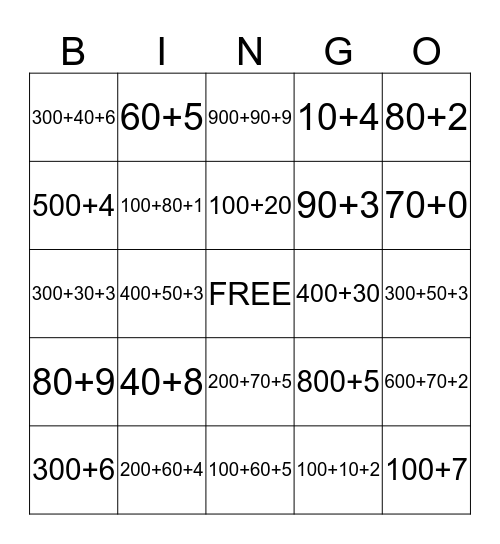# What is my number?This bingo card has a free space and 24 words: 40+8, 200+60+4, 100+20, 300+6, 80+2, 300+40+6, 500+4, 60+5, 400+30, 200+70+5, 90+3, 300+30+3, 800+5, 600+70+2, 100+80+1, 100+10+2, 300+50+3, 900+90+9, 70+0, 400+50+3, 100+7, 80+9, 10+4 and 100+60+5.

## Play Online